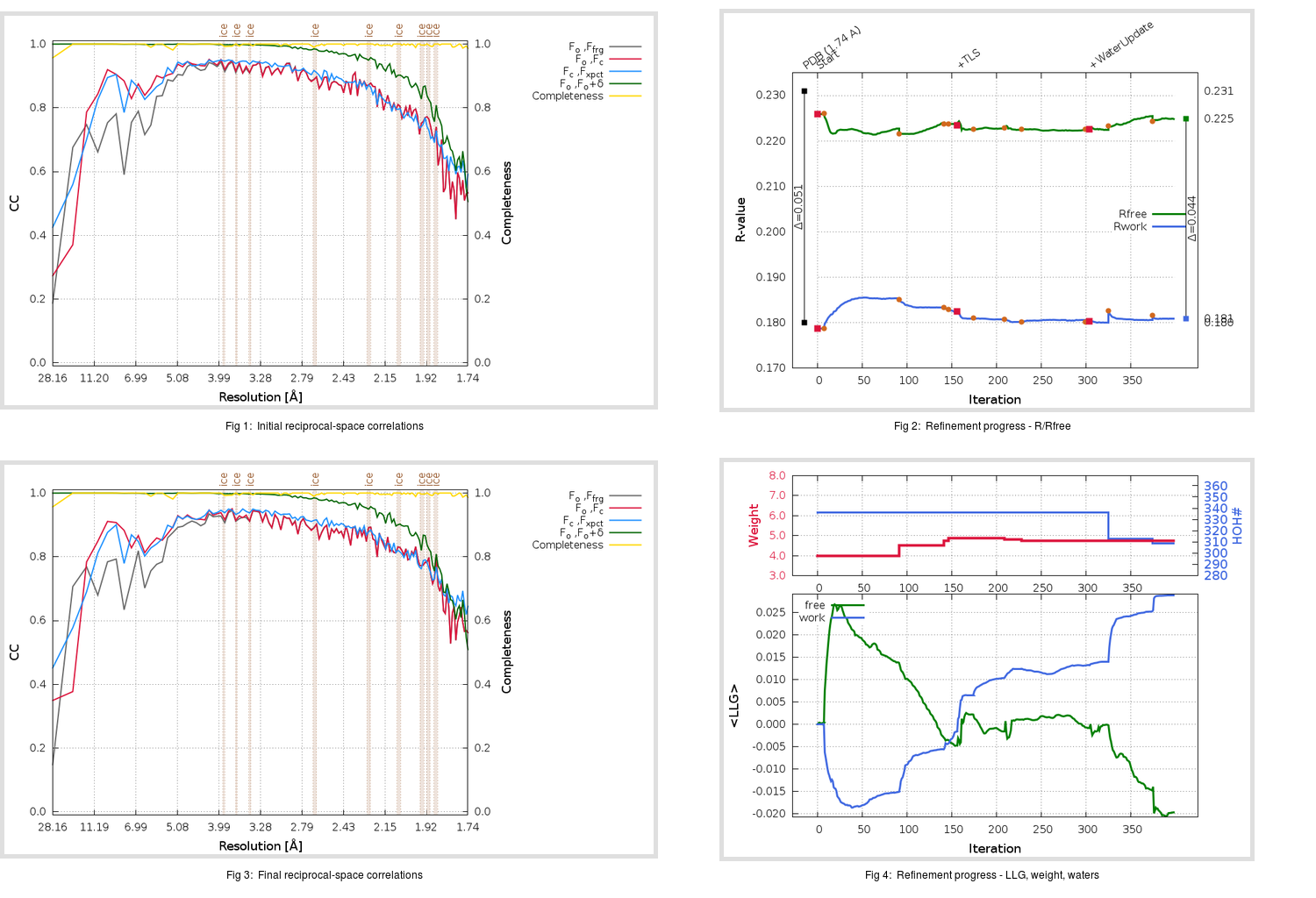Content:

```    Diffraction limits & principal axes of ellipsoid fitted to diffraction cut-off surface:
1.858         0.9663   0.0000   0.2574       a* + 0.015 c*
1.687         0.0000   1.0000   0.0000       b*
1.664        -0.2574   0.0000   0.9663      -0.544 a* + 0.839 c*
```

## Deposited

` `
 Date deposited Date data collection Resolution R, Rfree 20200315 20200305 1.74 0.1780 0.2310

Molprobity (CCP4 7.0 version) summary:

```Ramachandran outliers =   0.33 %
favored =  97.02 %
Rotamer outliers      =   0.38 %
C-beta deviations     =     0
Clashscore            =   5.50
RMS(bonds)            =   0.0131
RMS(angles)           =   1.82
MolProbity score      =   1.47
Resolution            =   1.74
R-work                =   0.1780
R-free                =   0.2310
```

```Number of waters      =   336

<B> (all atoms) =   27.08 ( sd =    9.94 ) for       2725 non-hydrogen atoms
<B>   (protein) =   25.46 ( sd =    8.77 ) for       2370 non-hydrogen atoms
<B>     (water) =   37.89 ( sd =   10.52 ) for        336 non-hydrogen atoms
<B>    (others) =   37.84 ( sd =   13.13 ) for         19 non-hydrogen atoms

B min/max       (all non-hydrogen atoms) =   14.46 /   79.64
B min/max   (protein non-hydrogen atoms) =   14.46 /   67.40
B min/max     (water non-hydrogen atoms) =   15.82 /   79.64
B min/max     (other non-hydrogen atoms) =   29.27 /   51.77
```

## BUSTER (re-)refinement

` `

Molprobity (CCP4 7.0 version) summary:

```Ramachandran outliers =   0.33 %
favored =  98.01 %
Rotamer outliers      =   1.14 %
C-beta deviations     =     0
Clashscore            =   2.75
RMS(bonds)            =   0.0113
RMS(angles)           =   1.56
MolProbity score      =   1.11
Resolution            =   1.74
R-work                =   0.1808
R-free                =   0.2248
```

```Number of waters      =   309

<B> (all atoms) =   26.37 ( sd =    9.09 ) for       2698 non-hydrogen atoms
<B>   (protein) =   24.73 ( sd =    7.52 ) for       2370 non-hydrogen atoms
<B>     (water) =   38.27 ( sd =   10.29 ) for        309 non-hydrogen atoms
<B>    (others) =   37.31 ( sd =   15.70 ) for         19 non-hydrogen atoms

B min/max       (all non-hydrogen atoms) =    6.75 /   67.72
B min/max   (protein non-hydrogen atoms) =    6.75 /   62.19
B min/max     (water non-hydrogen atoms) =   15.44 /   67.72
B min/max     (other non-hydrogen atoms) =   25.94 /   55.82
```

Refinement progression:Results:

` `
 File Remark 5RF5_aB_refine.01_03_refine.pdb.gz exact refinement commands are in header 5RF5_aB_refine.01_03_refine.mtz.gz including original deposited data and several re-refinement map coefficients 5RF5_aB_refine.01_03_BUSTER_model.cif.gz including any non-standard compound restraints 5RF5_aB_refine.01_03_BUSTER_refln.cif.gz